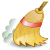# Dimensionality of space:timeThe content of this article requires cleaning up to meet OD's quality standards. Check the wiki best practices for guidelines on improving article and categorisation quality.

Category:Joe's observations Observations that support the notion that spacetime in nature does not exceed 3 orthogonal dimensions

##### NFCM (New Foundations for Classical Mechanics) summary - David Hestenes Ch. 1
• P. 3 reciprocal identity of k-blade and k-vector only holds for $\mathcal{G}_n, n \leq 3$
"The completely antisymmetrized product of k vectors $a_1, a_2 \ldots a_k$ generates a new entity $a_1 \wedge a_2 \wedge \ldots \wedge a_k$ called a k-blade. The integer k is called the step (or grade of the blade.* A linear combination of blades with the same step is called a k-vector. Therefore, every k-blade is a k-vector. The converse that every k-vector is a k-blade holds only in the geometric algebras $\mathcal{G}_n$ with $n \leq 3$." (Italics, mine)
• P. 8 Special and unique properties of i in 3-space, as unit pseudoscalar, as dual, as inverse space
"The properties of geometric algebra which are peculiar to the three-dimensional case are summarized in this section. They all derive from special properties of the pseudoscalar and duality in $\mathcal{G}_3$.
"The unit pseudoscalar for $\mathcal{G}_3$ is so important that the special symbol $i$ is reserved to denote it. This symbol is particularly apt because $i$ has the algebraic properties of a conventional unit imaginary. Thus, it satisfies the equations
(2.1) $i^2 = -1$
and
(2.2) $i\mathbf{a} = \mathbf{a}i$
for any vector $\mathbf{a}$. According to (2.2), the imaginary number $i$ commutes with vectors, just like the real numbers (scalars). It follows that $i$ commutes with every multivector in $\mathcal{G}_3$.
The algebraic properties (2.1) and (2.2) allow us to treat $i$ as if it were an imaginary scalar, but $i$ has other properties deriving from the fact that it is the unit pseudoscalar. In particular, $i$ relates scalars and vectors in $\mathcal{G}_3$ to bivectors and pseudoscalars by duality."
...
(2:4) $A = \alpha + \mathbf{a} + i\mathbf{b} + i\beta$
"This shows that $A$ has the formal algebraic structure of a complex scalar $\alpha + i\beta$ added to a complex vector $\mathbf{a} + i\mathbf{b}$. The algebraic advantages of the "complex expanded form" (2.4) are such that we shall use the form often."

To Do: fix maths formula numbering without using tables''To Do: fix maths formula size inconsistencies

What this means to me The discoveries in GA indicate that 3-space has logical properties and geometric interpretations that:

• seem to place special emphasis on the validity of 3-space as more 'natural' than higher n-spaces, since special identities in 3-space do not hold in higher spaces.
• the special geometric interpretation and operational function of the imaginary unit $i$ is reminiscent of its function in quaternion rotations. the analogy in GA is that it transforms vector objects, scalars, and pseudoscalars (spaces) into their duality complement. but this rotation is not in a hyperspace, but rather in an involute space (or inverse space). this says something about the nature of involute spaces - the transformation of a 3-space into an (apparent) scalar and the inverse transformation. this is analogous to the reciprocal geometry in RST.
##### Bott periodicity

To Do: find reference